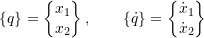### Approximate Methods for Multiple Degree of Freedom Systems: Kinetic and Potential Energies in Multiple Degree of Freedom in Systems

So far we have considered multiple degree of freedom systems and have shown how to find the natural frequencies and modes shapes associated with such systems, which is essentially an eigenvalue problem. Often we are only interested in the lowest (or possibly the largest) natural frequency in a system. There are methods that can be used to estimate these frequencies which do not entail finding all of the natural frequencies of a system. We will discuss two such methods in this section, Rayleigh’s Quotient and Dunkerley’s Formula. To begin with, however, we need to consider how we can represent kinetic and potential energies in multiple degree of freedom systems.

Consider an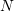degree of freedom system withcoordinates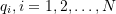describing some displaced configuration of the system. Each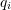may be a linear or angular displacement. Let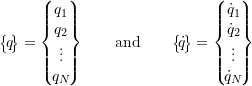represent the values of the coordinates and their associated derivatives. For the linear systems considered here it can be shown that the potential energy in the system is given by

(9.1)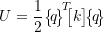where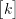is the stiffness matrix discussed earlier. Note that the potential energy is therefore zero at the equilibrium position. Similarly, the kinetic energy is given by

(9.2)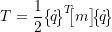where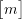is the mass matrix.

#### EXAMPLE

At some instant in time, the masses in the system shown below are in positions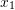and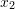and have velocities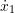and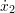, respectively.

a) Determine the potential and kinetic energies in the system in terms of,,, andfrom first principles.

b) Show that the potential and kinetic energies can also be expressed as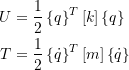where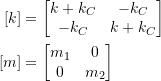and